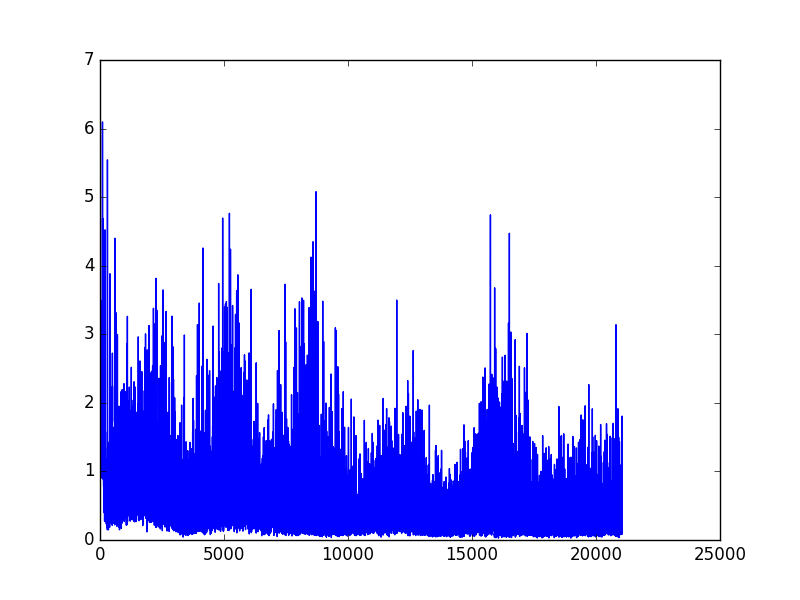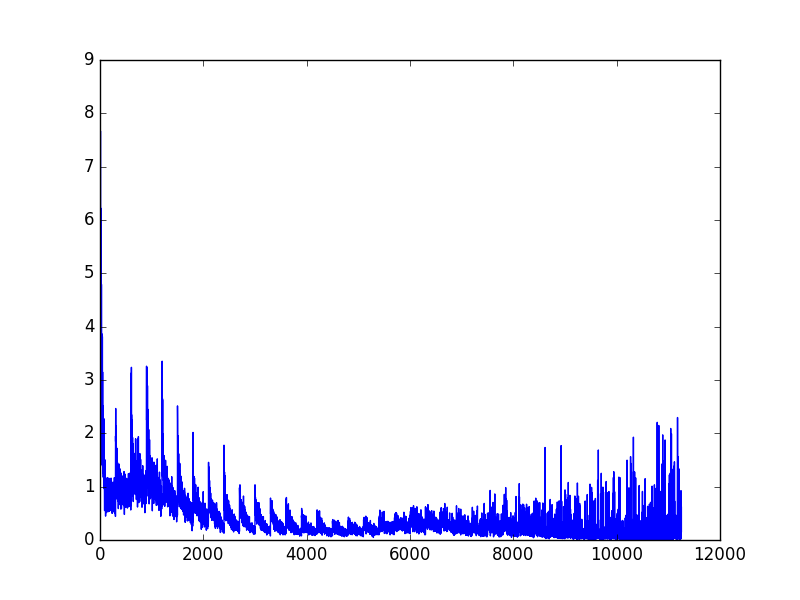# OpenAI gym 环境库

## 安装 gym ¶

# python 2.7, 复制下面
$pip install gym # python 3.5, 复制下面$ pip3 install gym


# MacOS:
$brew install cmake boost boost-python sdl2 swig wget # Ubuntu 14.04:$ apt-get install -y python-numpy python-dev cmake zlib1g-dev libjpeg-dev xvfb libav-tools xorg-dev python-opengl libboost-all-dev libsdl2-dev swig


# python 2.7, 复制下面
$pip install gym[all] # python 3.5, 复制下面$ pip3 install gym[all]


## CartPole 例子 ¶

import gym
from RL_brain import DeepQNetwork

env = gym.make('CartPole-v0')   # 定义使用 gym 库中的那一个环境
env = env.unwrapped # 不做这个会有很多限制

print(env.action_space) # 查看这个环境中可用的 action 有多少个
print(env.observation_space)    # 查看这个环境中可用的 state 的 observation 有多少个
print(env.observation_space.high)   # 查看 observation 最高取值
print(env.observation_space.low)    # 查看 observation 最低取值


# 定义使用 DQN 的算法
RL = DeepQNetwork(n_actions=env.action_space.n,
n_features=env.observation_space.shape,
learning_rate=0.01, e_greedy=0.9,
replace_target_iter=100, memory_size=2000,
e_greedy_increment=0.0008,)

total_steps = 0 # 记录步数

for i_episode in range(100):

# 获取回合 i_episode 第一个 observation
observation = env.reset()
ep_r = 0
while True:
env.render()    # 刷新环境

action = RL.choose_action(observation)  # 选行为

observation_, reward, done, info = env.step(action) # 获取下一个 state

x, x_dot, theta, theta_dot = observation_   # 细分开, 为了修改原配的 reward

# x 是车的水平位移, 所以 r1 是车越偏离中心, 分越少
# theta 是棒子离垂直的角度, 角度越大, 越不垂直. 所以 r2 是棒越垂直, 分越高

x, x_dot, theta, theta_dot = observation_
r1 = (env.x_threshold - abs(x))/env.x_threshold - 0.8
reward = r1 + r2   # 总 reward 是 r1 和 r2 的结合, 既考虑位置, 也考虑角度, 这样 DQN 学习更有效率

# 保存这一组记忆
RL.store_transition(observation, action, reward, observation_)

if total_steps > 1000:
RL.learn()  # 学习

ep_r += reward
if done:
print('episode: ', i_episode,
'ep_r: ', round(ep_r, 2),
' epsilon: ', round(RL.epsilon, 2))
break

observation = observation_
total_steps += 1
# 最后输出 cost 曲线
RL.plot_cost()## MountainCar 例子 ¶

import gym
from RL_brain import DeepQNetwork

env = gym.make('MountainCar-v0')
env = env.unwrapped

print(env.action_space)
print(env.observation_space)
print(env.observation_space.high)
print(env.observation_space.low)

RL = DeepQNetwork(n_actions=3, n_features=2, learning_rate=0.001, e_greedy=0.9,
replace_target_iter=300, memory_size=3000,
e_greedy_increment=0.0001,)

total_steps = 0

for i_episode in range(10):

observation = env.reset()
ep_r = 0
while True:
env.render()

action = RL.choose_action(observation)

observation_, reward, done, info = env.step(action)

position, velocity = observation_

# 车开得越高 reward 越大
reward = abs(position - (-0.5))

RL.store_transition(observation, action, reward, observation_)

if total_steps > 1000:
RL.learn()

ep_r += reward
if done:
get = '| Get' if observation_ >= env.unwrapped.goal_position else '| ----'
print('Epi: ', i_episode,
get,
'| Ep_r: ', round(ep_r, 4),
'| Epsilon: ', round(RL.epsilon, 2))
break

observation = observation_
total_steps += 1

RL.plot_cost()